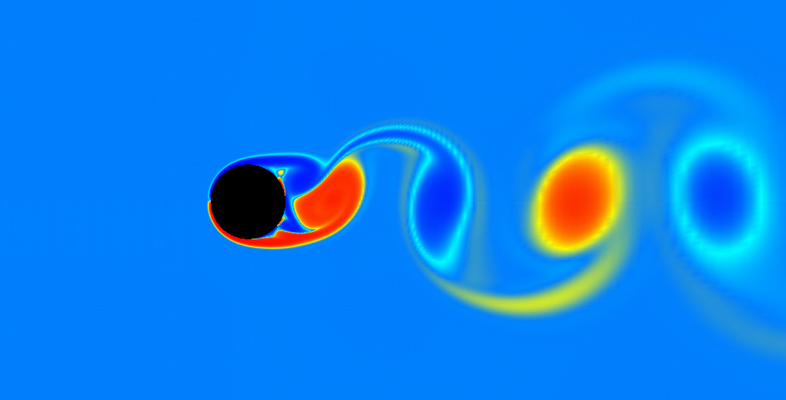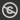Science, Maths & Technology
Free course

# Kinematics of fluids## Course reviews

This free course examines the basic kinematics of two-dimensional fluid flows. Section 1 introduces the diﬀerential equations for pathlines and streamlines. Section 2 introduces a scalar ﬁeld, called the stream function, which for an incompressible ﬂuid provides an alternative method of modelling the ﬂow and ﬁnding the streamlines. Sections 2 and 3 derive the stream functions for several simple two-dimensional ﬂow types (the uniform ﬂow, source, doublet and vortex), and suitable combinations of these are used to model more complicated ﬂows. Section 4 introduces the idea of diﬀerentiation following the motion, and Euler’s equation is developed in Section 5.

## Course learning outcomes

After studying this course, you should be able to:

• derive the pathlines and the streamlines in Cartesian and polar form from a velocity field
• derive the stream function from a velocity field
• model different flows from a combination of uniform flows, sources, sinks and doublets
• use the continuity equation to determine whether an inviscid flow is incompressible
• understand how Euler's equation is derived, what it represents, and use it to find the pressure distribution from a velocity.

First Published: 09/03/2018

Updated: 23/07/2019

Skip Rate and Review